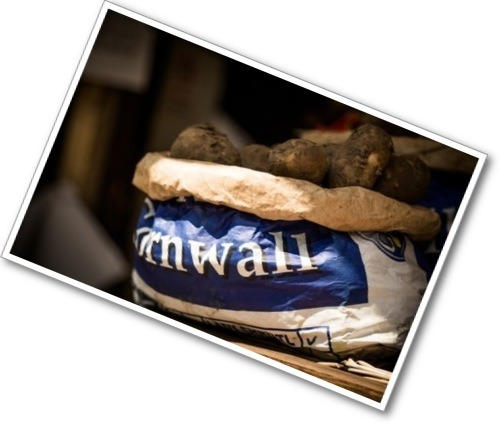Author:
Subject:
Ratios and Proportions
Material Type:
Lesson Plan
Level:
Middle School
6
Provider:
Pearson
Tags:
6th Grade Mathematics, Cooking, Fractions, Ingredients
Language:
English
Media Formats:
Text/HTML

# Gallery Problems# Gallery Overview

Allow students who have a clear understanding of the content in the unit to work on Gallery problems of their choosing. You can use this time to provide additional help to students who need review of the unit's concepts or to assist students who may have fallen behind on work.

# Gallery Description

## Stew Recipe

Students use fraction operations to help Molly figure out if she has enough potatoes to make stew for all the guests at her party.

## Multiply or Divide?

Students match descriptions of situations to multiplication and division situations.

## Card Sort

Students find the diagram, expression, and answer that match given word problems.

## Complex Fractions

Students learn about complex fractions and how they are useful for dividing fractions.

# Stew Recipe

1. $36×\frac{3}{4}=27$
Possible answer: Molly will need 27 pounds of potatoes to serve all 36 people.
2. $24÷\frac{3}{4}=32$
Possible answer: Molly has enough potatoes to serve 32 people.
3. No, Molly needs 3 more pounds of potatoes.

# Stew Recipe

Molly’s recipe for stew says to use $\frac{3}{4}$ pound of potatoes per person. Molly has 24 pounds of potatoes. Molly wonders if she can make enough stew to serve all 36 people at her party.

1. Calculate $36×\frac{3}{4}$. What information can Molly get from the answer?
2. Calculate $24÷\frac{3}{4}$. What information can Molly get from the answer?
3. Will Molly have enough potatoes? If not, how many more pounds will she need?

# Multiply or Divide?

1. a) $3\frac{1}{2}$ ÷ $\frac{3}{4}$

b) $3\frac{1}{2}$ × $\frac{3}{4}$

2. a) $2\frac{2}{3}$ × $\frac{1}{3}$

b) $2\frac{2}{3}$ ÷ $\frac{1}{3}$

3. a) Answers will vary. Possible answer: The number of people she could feed if she gave each person $\frac{1}{8}$ pound.

b) Answers will vary. Possible answer: The number of pounds she would eat if she ate $\frac{1}{8}$ of the pasta salad.

# Multiply or Divide?

1. Daniela has a ribbon $3\frac{1}{2}$ feet long. Consider the following expressions:

$3\frac{1}{2}÷\frac{3}{4}$
$3\frac{1}{2}×\frac{3}{4}$

a) Which expression represents the number of pieces she would have if she cut the ribbon into $\frac{3}{4}$-foot pieces?

b) Which expression gives the length of $\frac{3}{4}$ of the ribbon in feet?

2. A recipe calls for $2\frac{2}{3}$ cups of flour. Consider the following expressions:

$2\frac{2}{3}÷\frac{1}{3}$
$2\frac{2}{3}×\frac{1}{3}$

a) Which expression represents the amount of flour in $\frac{1}{3}$ of a recipe?

b) Which expression represents the number of times a $\frac{1}{3}$ cup measure would need to be filled to measure the flour?

3. Ji Young made $5\frac{1}{2}$ pounds of pasta salad. Consider the expressions below.

$5\frac{1}{2}÷\frac{1}{8}$
$5\frac{1}{2}×\frac{1}{8}$

a) Tell what the expression $5\frac{1}{2}÷\frac{1}{8}$ could represent in this situation.

b) Tell what the expression $5\frac{1}{2}×\frac{1}{8}$ could represent in this situation.

# Card Sort

Explanations will vary.

# Card Sort

Work with a partner.

Take turns finding the expression card, diagram card, and answer card that matches each problem.

Explain to your partner how you know that the cards match the problem.

Your partner should either agree with your explanation or challenge it if he or she thinks your explanation is not correct, clear, or complete.

For each matched set, explain what the solution card represents.

INTERACTIVE: Card Sort

# Complex Fractions

1. $\frac{\frac{3}{4}}{\frac{5}{6}}$

2. $\frac{\frac{3}{4}}{\frac{5}{6}}×\frac{12}{12}=\frac{9}{10}$

3. $\frac{9}{10}×\frac{5}{6}=\frac{45}{60}=\frac{3}{4}$

4. $2\frac{1}{3}÷\frac{1}{2}=\frac{\frac{7}{3}}{\frac{1}{2}}=\frac{\frac{7}{3}}{\frac{1}{2}}×\frac{6}{6}=\frac{14}{3}=4\frac{2}{3}$

# Complex Fractions

A complex fraction is a fraction whose numerator and/or denominator contain fractions. Here are some examples of complex fractions:

$\frac{3}{\frac{4}{5}}$

$\frac{\frac{4}{7}}{3}$ $\frac{\frac{2}{3}}{\frac{5}{8}}$

You can change a complex fraction to a regular fraction by multiplying it by 1 in a form that “clears” the fractions.

$\frac{\frac{2}{3}}{\frac{5}{8}}=\frac{\frac{2}{3}}{\frac{5}{8}}×\frac{24}{24}=\frac{\frac{48}{3}}{\frac{120}{8}}=\frac{16}{15}$

You can use this idea to divide fractions.

Consider the problem $\frac{3}{4}$ ÷ $\frac{5}{6}$.

1. Write the division problem as a complex fraction.

2. Multiply the complex fraction by 1 in a form that will clear the fractions in the numerator and denominator, giving a regular fraction. The result is the solution to $\frac{3}{4}$ ÷ $\frac{5}{6}$.

3. Use multiplication to check that the result from Part 2 is the solution to the division problem.

4. Use the complex fraction method to find 2$\frac{1}{3}$ ÷ $\frac{1}{2}$. (You will need to write 2$\frac{1}{3}$ as a fraction.)